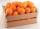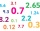# Lemonade

How many 1/4 cup servings are in 2 and 1/3 cups of lemonade?

Result

n =  9

#### Solution:Leave us a comment of example and its solution (i.e. if it is still somewhat unclear...):

Showing 0 comments:Be the first to comment!#### To solve this example are needed these knowledge from mathematics:

Need help calculate sum, simplify or multiply fractions? Try our fraction calculator.

## Next similar examples:

1. Crates 2One crate will hold 50 oranges. If Bob needs to ship 932 oranges, how many crates will he need?
2. Fractions 4How many 2/3s are in 6?
3. The resultHow many times I decrease the number 1632 to get the result 24?
4. Lengths of the poolMiguel swam 6 lengths of the pool. Mat swam 3 times as far as Miguel. Lionel swam 1/3 as far as Miguel. How many lengths did mat swim?
5. Product of two fractionsProduct of two fractions is 9 3/5 . If one of the fraction is 9 3/7. Find the other fraction.
6. Pizza 5You have 2/4 of a pizza and you want to share it equally between 2 people how much pizza does each person get?
7. ChocolateChildren break chocolate first to third and then every part of another half. What kind got each child? Draw a picture. What part would have received if each piece have halved?
8. Unit rateFind unit rate: 6,840 customers in 45 days
9. Cake 71/3 of a cake shared with 4 people. What share of the whole cake has each people?
10. What is one thirdWhat is 1/3 as a decimal? Give your answer rounded to 2 decimal places.
11. Round it0.728 round to units, tenths, hundredths.
12. One-third 2One-third of the people in a barangay petitioned the council to allow them to plant in vacant lots and another 1/5 of the people petitioned to have a regular garbage collection. What FRACTION of the barangay population made the petition?
13. ZdeněkZdeněk picked up 15 l of water from a 100-liter full-water barrel. Write a fraction of what part of Zdeněk's water he picked.
14. Mixed2improperWrite the mixed number as an improper fraction. 166 2/3
15. Fraction and a decimalWrite as a fraction and a decimal. One and two plus three and five hundredths
16. Fraction to decimalWrite the fraction 3/22 as a decimal.
17. In fractionsAn ant climbs 2/5 of the pole on the first hour and climbs 1/4 of the pole on the next hour. What part of the pole does the ant climb in two hours?